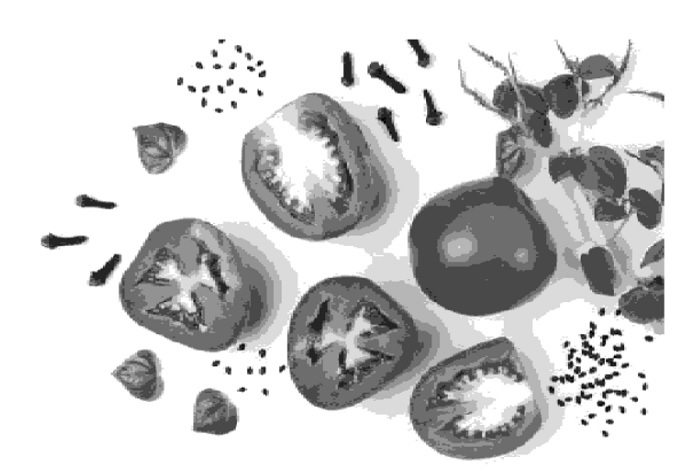# Gray Level Co-occurrence Matrix in MATLAB

In digital image processing, the gray level co-occurrence matrix, also known as GLCM, is a statistical method employed for holding the spatial relationship between pairs of pixels in a digital image.

The gray level co-occurrence is a method of representing the way in which different combinations of pixel intensities are arranged within the image. It is mainly used to specify the texture property of an image and provide the information about the patterns, variations, and structures occurring in the pixel values within spatial regions of the image.

## What is Gray Level Co-Occurrence Matrix?

As stated above, the gray level co-occurrence matrix (GLCM) is a statistical method that provides information about relationship between pixel intensities in a specified local region of an image.

The gray level co-occurrence matrix is basically a square matrix whose elements represent the frequency of a pixel occurrence in an image. This technique is quite useful in obtaining information about texture and patterns present in an image in image processing applications.

## How to Calculate Gray Level Co-occurrence Matrix in MATLAB?

MATLAB provides a built-in function 'geaycomatrix' to calculate the gray level co-occurrence matrix from an image.

This function can have the following three syntaxes:

glcm = graycomatrix(I)
glcm = graycomatrix(I, Name, Value)
[glcm, SI] = graycomatrix(___)


We will cover all these syntaxes in the upcoming sections of this article. Before that let's get an idea about the step-by-step process of calculating the gray level co-occurrence matrix using MATLAB.

## Process of Calculating Gray Level Co-occurrence Matrix

The step-by-step process for calculating the gray level co-occurrence matrix from an image using MATLAB is explained below:

Step (1) – Read the input image and convert it to gray scale version.

Step (2) – Define the parameters such as distance between pixel pairs, angle, etc. for calculating the gray level co-occurrence matrix.

Step (3) – Compute the gray level co-occurrence matrix using the 'graycomatrix' function.

This gray level co-occurrence matrix then can be used to analyze the texture and pattern properties of the image.

Now, let us practically understand how to create a gray level co-occurrence matrix from an image in MATLAB.

## Calculate Gray Level Co-occurrence Matrix using Default Parameters

In MATLAB, we can use the following syntax of the 'graycomatrix' function to create the gray level co-occurrence matrix (GLCM) from image with default parameters.

glcm = graycomatrix(I);


Where, I is the input gray scale image.

### Example

Let us now consider an example program to demonstrate the implementation of this syntax in MATLAB programming.

% MATLAB program to create GLCM with default parameters

% Convert the input image to gray scale image
I = rgb2gray(img);

% Compute GLCM with default parameters
glcm = graycomatrix(I);

% Display the GLCM
disp('The GLCM for the input image is:');
disp(glcm);


### Output

The GLCM for the input image is:
33	129	41	14	1	0	0	0
135	2874	778	223	84	24	3	0
31	791	22294	3199	329	125	35	1
15	174	3236	28009	3252	394	152	16
3	106	311	3128	14310	2148	317	74
1	42	140	485	1775	12637	2085	200
0	6	59	186	513	1594	24010	2485
0	0	6	40	155	484	2289	136872


## Calculate Gray Level Co-occurrence Matrix using Specified Parameters

In MATLAB, the following syntax of the 'graycomatrix' function is used to calculate the gray level co-occurrence matrix using specified parameters.

glcm = graycomatrix(I, Name, Value, …);


In this syntax, we specify the parameters by using the Name-Value pairs.

### Example

The following example demonstrates the practical implementation of this syntax in MATLAB programming.

% MATLAB program to create GLCM with specified parameters

% Convert the input image to gray scale image
I = rgb2gray(img);

% Compute GLCM with default parameters
glcm = graycomatrix(I, 'Offset', [2 1], 'NumLevels', 7);

% Display the GLCM
disp('The GLCM for the input image is:');
disp(glcm);


### Output

The GLCM for the input image is:
53	193	33	26	34	53	54
206	4087	1611	406	243	190	283
26	1607	32291	5636	1006	486	352
24	413	5511	20083	3230	844	604
29	236	1051	2957	11465	2442	768
49	201	555	808	1938	20689	3416
57	284	410	811	1073	2974	139777


## Calculate Gray Level Co-occurrence Matrix Returning Scaled Image

In MATLAB, the following syntax of the 'graycomatrix' function is used to calculate the gray level co-occurrence matrix that returns a scaled image.

[glcm, SI] = graycomatrix(___);


Here, the variable 'SI' is the scaled image which is basically the input image normalized to the range [1, NumLevels].

## Example

Let us see an example to understand the implementation of this syntax in MATLAB programming.

% MATLAB program to create GLCM returning scaled image

% Convert the input image to gray scale image
I = rgb2gray(img);

% Compute GLCM with default parameters
[glcm, SI] = graycomatrix(I);

% Display the GLCM and scaled image
disp('The GLCM for the input image is:');
disp(glcm);
disp('The scaled image is:');
imshow(rescale(SI));


### Output

The GLCM for the input image is:
The GLCM for the input image is:
33	129	41	14	1	0	0	0
135	2874	778	223	84	24	3	0
31	791	22294	3199	329	125	35	1
15	174	3236	28009	3252	394	152	16
3	106	311	3128	14310	2148	317	74
1	42	140	485	1775	12637	2085	200
0	6	59	186	513	1594	24010	2485
0	0	6	40	155	484	2289	136872


The scaled image is:## Conclusion

This is all about creating a gray level co-occurrence matrix (GLCM) in MATLAB. For this, MATLAB provides a built-in function 'graycomatrix' that allows to calculate the comatrix from an image to analyze the texture characteristics of the image.

In this tutorial, we have explained the concept of gray level co-occurrence matrix, its significance in image processing and practically examples to understand how to calculate the GLCM for an image using MATLAB.

Updated on: 07-Sep-2023

43 Views